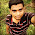## Java program to find smallest number between N number

Java program to find smallest number between 10 number

Java program to find smallest number between 5 number

Java program to find smallest number between 21 number

Java program to find minimum number between N number

Java program to find lowest number between N number

Java program to find smallest number without using array

Program

import java.util.Scanner;

class group{
public static void main(String argn[]){
Scanner data = new Scanner(System.in);
int num, i, temp, small;
System.out.print("Enter number you want to enter:");
num = data.nextInt();
System.out.println("Enter " +num+ " number");
small = data.nextInt();
for (i=1; i < num; i++)
{
temp = data.nextInt();
if(temp > small)
continue;
else
// smallest number
small = temp;

}

System.out.println("Smallest Number is "+small);

}
}

Output:-

Enter number you want to enter:10
Enter 10 number
584
114
1444
144
114
552
116
446
149
181
Smallest Number is 114

Learn Java:-
Java codes

Java Programs:-
List of Java Programs

1.I got 2 errors. could you please explain why? this is the code.
import java.util.Scanner;

class smallest
{

public static void main(String args[])
{
Scanner data = new Scanner(System.in);

int num, i, temp, small;
System.out.println("How many numbers do you want to enter?");
num = data.nextInt();
if (num>1)
{
System.out.println("Enter the number:");
}
else
{
System.out.println("Enter the numbers:");
}
small = data.nextInt;
for(i = 1; i < num; i++)
{
temp = data.nextInt;
if(temp > small)
{
continue;
}
else
{
small = temp;
}
}
System.out.println("The smallest number is: " + small);
}
}

E:\funny\java>javac smallest.java
smallest.java:20: error: cannot find symbol
small = data.nextInt;
^
symbol: variable nextInt
location: variable data of type Scanner
smallest.java:23: error: cannot find symbol
temp = data.nextInt;
^
symbol: variable nextInt
location: variable data of type Scanner
2 errors

2.You forget put () write like this
temp = data.nextInt();

3.It works and good work